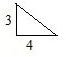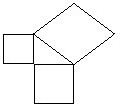# Explaining the Proof of the Pythagorean Theorem: Eighth Grade Math Lesson

Page content

In this lesson work with the students to construct similar figures on the three sides of a right triangle. Find the areas of the similar figures. Compare the sum of the area of the similar figures constructed on the right triangle legs to the area of the similar figure constructed on the hypotenuse.

Learning Objective: The lesson is aligned to the Common Core State Standards for Mathematics - 8.G.6 Geometry – Explain a proof of the Pythagorean Theorem and its converse.

Materials Required: dot paper, graph paper, calculator

## Lesson Procedure:

**

Identifying the Parts of a Right Triangle

Draw a right triangle on dot paper and label the parts of the right triangle.Finding the Area of Similar Figures Drawn on the Sides of a Right Triangle

1. On dot paper, draw a right triangle with one leg measuring 3 units and the other leg 4 units.

2. On the leg measuring 3 units, draw a square with the four sides measuring 3 units. What is the area of the square?

3. On the leg measuring 4 units, draw a square with the four sides measuring 4 units. What is the area of the square?

4. On the hypotenuse, draw a square. To find the area of this square, count the number of square units inside the square on the dot paper. What is the area of the square?5. The areas of the squares on the two legs are _____ square units and _____ square units. The area of the square on the hypotenuse is _____ square units.

6. Write the equation to show the relationship between the sum of the area of the squares constructed on the legs of a right triangle to the area of the square constructed on the hypotenuse of the right triangle.

7. The area of the square constructed on the hypotenuse is _____ square units. The measure of the sides of the square is the square root of the area. What is the measure of the hypotenuse?

1. 9 square units
2. 16 square units
3. 25 square units
4. The areas of the squares on the two legs are 9 square units and 16 square units. The area of the square on the hypotenuse is 25 square units.
5. 9 + 16 = 25
6. 25, 5

## Individual or Group Work:

Individual or Group Work:

1. On dot paper, draw a right triangle with legs measuring 7 and 10 units.

a. Draw the hypotenuse.
b. On the leg measuring 7 units, draw a square with the four sides measuring 7 units. What is the area of the square?
c. On the leg measuring 10 units, draw a square with the four sides measuring 10 units. What is the area of the square?
d. On the hypotenuse, draw a square. To find the area of this square, count the number of square units inside the square on the dot paper. What is the area of the square?
e. The areas of the squares on the two legs are _____ square units and _____ square units. The area of the square on the hypotenuse is _____ square units.
f. Write an equation to show the relationship between the sum of the area of the squares constructed on the legs of a right triangle to the area of the square constructed on the hypotenuse of the right triangle.
g. The area of the square constructed on the hypotenuse is _____ square units. The measure of the sides of the square is the square root. What is the measure of the hypotenuse? Calculate the measure to the nearest tenth.

2. On dot paper, draw a right triangle. Construct similar figures on three sides of the right triangle.

a. Write a numerical equation to show the relationship between the areas of the similar figures.
b. Write an algebraic equation to show the relationship between the areas of the similar figures.

3. If similar figures are constructed on the sides of a right triangle, then the area of the figure on the hypotenuse is equal to __________________________________________________.

4. The measure of the hypotenuse __________ is equal to the __________ of the measure of the legs __________.

5. The measures of the three sides of a figure are 4 units, 8 units, and 12 units. Is the figure a right triangle? Explain your answer.

6. The measures of two legs of a right triangle are 6 cm and 9 cm. What is the measure of the hypotenuse to the nearest tenth? Explain how you found your answer.

Students should be able to generalize that the square of the sum of the areas of similar figures drawn on the legs of a right triangle equal the area of the similar figure drawn on the hypotenuse. The students should be able to express this relationship with the algebraic equation a2 + b2 = c2 and identify this relationship as the Pythagorean Theorem, a2 + b2 = c2.

1.
b. 49 square units
c. 100 square units
d. 149 square units
e. 49 square units and 100 square units; 149 square units
f. 7^2 + 〖10〗^2 = 149
g. 149 square units; 12.2

2. Answers will vary, a^2 + b^2= c^2

3. If similar figures are constructed on the sides of a right triangle, then the area of the figure on the hypotenuse is equal to the sum of the areas of the figures on the legs.

4. The measure of the hypotenuse squared is equal to the sum of the measure of the legs squared.

5. The figure is not a right triangle because the sum of the measure of the legs squared does not equal the hypotenuse square. 42+ 82 ≠ 12, 16 + 64 ≠ 144

6. 10.8 cm because 62 + 92 = 36 + 81 = 117, √117 ≈10.8

## This post is part of the series: Teaching the Pythagorean Theorem.

This series of lesson plans is intended for an eighth grade math class. Find a series of lessons that will teach your students about the Pythagorean theorem.